Test: Operations On Sets

# Test: Operations On Sets

Test Description

## 10 Questions MCQ Test Mathematics (Maths) Class 11 | Test: Operations On Sets

Test: Operations On Sets for Commerce 2022 is part of Mathematics (Maths) Class 11 preparation. The Test: Operations On Sets questions and answers have been prepared according to the Commerce exam syllabus.The Test: Operations On Sets MCQs are made for Commerce 2022 Exam. Find important definitions, questions, notes, meanings, examples, exercises, MCQs and online tests for Test: Operations On Sets below.
Solutions of Test: Operations On Sets questions in English are available as part of our Mathematics (Maths) Class 11 for Commerce & Test: Operations On Sets solutions in Hindi for Mathematics (Maths) Class 11 course. Download more important topics, notes, lectures and mock test series for Commerce Exam by signing up for free. Attempt Test: Operations On Sets | 10 questions in 10 minutes | Mock test for Commerce preparation | Free important questions MCQ to study Mathematics (Maths) Class 11 for Commerce Exam | Download free PDF with solutions
 1 Crore+ students have signed up on EduRev. Have you?
Test: Operations On Sets - Question 1

### Which of the following two sets are disjoint?

Detailed Solution for Test: Operations On Sets - Question 1

{1,3,5}&{2,4,6} are disjoint sets.

Explanation:

In {1,3,5} & {1,3,6} 1 and 3 is the same numbers.

In {1,2,3} & {1,2,3} 1,2 and 3 is the same numbers.

In {1,3,5} & {2,3,4} 3 is the same number.

In {1,3,5} & {2,4,6} not any numbers are same.

Test: Operations On Sets - Question 2

### The Shaded region in the following figure illustrates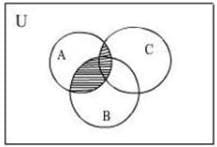Detailed Solution for Test: Operations On Sets - Question 2

First which region is over which region Then We will see that A is on the B so A intersection B and after C is on the A so, A intersection C after that we have to take all intersection part so A intersection B is Union with A intersection C.

The shaded region represents (A ∩ B) ∪ (A ∩ C).

Test: Operations On Sets - Question 3

### Shaded region in the following figure illustrates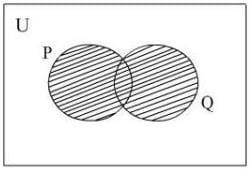Detailed Solution for Test: Operations On Sets - Question 3

P U Q means P Union Q In set theory, the union (denoted by ∪) of a collection of sets is the set of all elements in the collection. It is one of the fundamental operations through which sets can be combined and related to each other.

Test: Operations On Sets - Question 4

If the sets A and B are defined as= {(xy) : ex∈ R}; = {(xy) : x,∈ R}, then

Detailed Solution for Test: Operations On Sets - Question 4

Since,ex andx do not meet for any∈ R∩ φ.

Test: Operations On Sets - Question 5

The intersection of the sets {1, 2, 5} and {1, 2, 6} is the set ______

Detailed Solution for Test: Operations On Sets - Question 5

The intersection of the sets A and B, is the set containing those elements that are in both A and B.

Test: Operations On Sets - Question 6

From the following Venn diagram, A ∩ (B ∪ C) is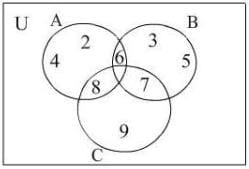Detailed Solution for Test: Operations On Sets - Question 6

Correct Answer :- B

Explanation:- B = {3,5,6,7}   C = {7,8,9}

A = {2,4,6,8}

A⋂(B⋂C) = {6,8}

Test: Operations On Sets - Question 7

If= {x is a multiple of 3} and= {x is a multiple of 5}, then A - B is

Detailed Solution for Test: Operations On Sets - Question 7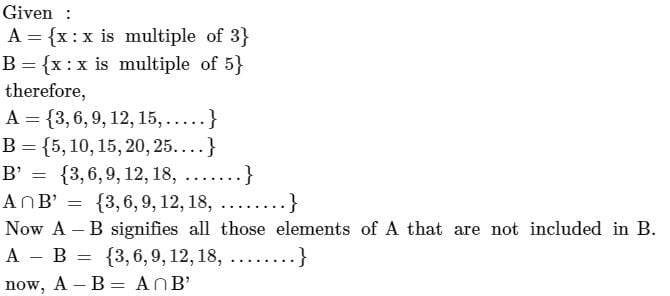Test: Operations On Sets - Question 8

From the following venn diagram, A ∩ B is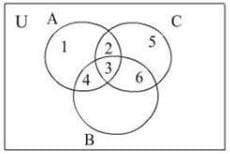Detailed Solution for Test: Operations On Sets - Question 8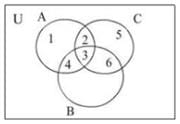A ∩ B = {4, 3}

Test: Operations On Sets - Question 9

In probability, the event ‘A or B’ can be associated with set:

Detailed Solution for Test: Operations On Sets - Question 9

Probability of event A or B

The probability that Events A and B both occur is the probability of the intersection of A and B. The probability of the intersection of Events A and B is denoted by P(A ∩ B). If Events A and B are mutually exclusive, P(A ∩ B) = 0. The probability that Events A or B occur is the probability of the union of A and B.

Test: Operations On Sets - Question 10

If A and B are two given sets, then∩ (∩ B)' is equal to

## Mathematics (Maths) Class 11

156 videos|171 docs|132 tests
 Use Code STAYHOME200 and get INR 200 additional OFF Use Coupon Code
Information about Test: Operations On Sets Page
In this test you can find the Exam questions for Test: Operations On Sets solved & explained in the simplest way possible. Besides giving Questions and answers for Test: Operations On Sets, EduRev gives you an ample number of Online tests for practice

## Mathematics (Maths) Class 11

156 videos|171 docs|132 tests